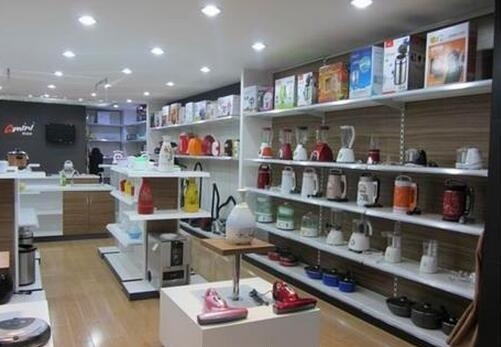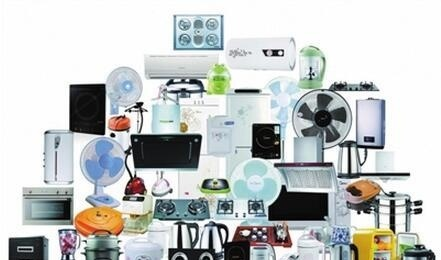|

# 小家电有哪些 那些成了你家里的僵尸小家电

搭乘年底家电促销的顺风车，小家电成为家电卖场、网购平台的热卖产品。“现在的年轻人都很注重生活品质，加上快过年了，很多人要准备给父母、给家人的礼物，各种功能的小家电现在卖得很火。”在郑州市经三路上一家电卖场，销售人员周先生刚刚送走了一对年轻顾客，他们选购了一台豆浆机，准备过年回家带给父母。周先生介绍，应季家电中电磁炉、加湿器、电暖气、豆浆机等小家电非常受欢迎，价格在100~500元的产品销量最好。在网购平台上，扫地机器人、电烤箱、微波炉、原汁机、面包机等产品，成为热门产品。一台价格为699元的原汁机，在其旗舰店的月销量有700多台。

然而，在销售一片火热的状态下，也有人发出了不同的声音，认为很多小家电“买时萌萌哒，过气蔫蔫哒”。有专业机构近日发布了中国城市职业女性家庭煮食小家电研究，数据显示小家电的闲置率在70%左右，其中，酸奶机和面包机闲置率最高。

探究

是“神器”还是“鸡肋”?

不得不说，这是个很奇特的现象。一方面，有人发声“唱衰”小家电，认为其实用性不强，闲置率高，不值得购买;另一方面，还有源源不断的人涌入这个市场，出手为自己添置小家电。

究竟是“神器”还是“鸡肋”?不如听听他们的故事吧。

故事

打入冷宫豆芽机

这几年，食品安全问题频发，人们对“什么能吃”充满疑问。为了能吃到放心菜，王女士花了不到200元，网购了一台豆芽机。她的想法是，与其吃外面“来路不明”的菜，不如自己在家生产。

豆芽机送到家，她很是新鲜了一阵子。插上电、加满水，三四天之后就能收获白白胖胖的豆芽，王女士觉得很不错，下厨的频率也高了很多。可豆芽菜再好，也不能天天吃，不到两个月，她的豆芽机就待在厨房的架子上落灰了，后来干脆放进了地下室，再也没用过。

点评：

对于很多年轻人来说，酸奶机、豆芽机、煮蛋器这样的厨房小家电，就像小时候玩福窝用到的玩具。赶时髦或者一时心血来潮买回家，用上几次也就失去了兴趣。毕竟，这个时代有太多新鲜好玩的东东随时吸引着人们的注意力。

故事

漂洋过海面条机

洛阳姑娘小刘远渡重洋到澳大利亚深造。2013年，她在澳洲的灿烂阳光下披上嫁衣，组建了自己的小家庭。

作为一个彻头彻尾的北方姑娘，她常常怀念自己的家乡饭——面条。但在遥远的澳洲，想吃到一碗新鲜的手擀面并不容易。也只有父母最懂女儿，不久前，小刘的父母出国探亲，一台自动面条机也跟着二老漂洋过海来到澳洲。

粗面、细面、龙须面，宽面、窄面、空心面，父母在她身边的这段时间，小刘可过足了面条瘾，微信朋友圈里不发别的，净是一碗一碗的爱心面，体重也直线上升。

点评：

小家电的基本功能之一，是帮主人完成一些手工做不到的操作。然而在小刘这里，这台面条机已经不仅仅是一件小家电，而被赋予了更深刻的含义——爱。父母之爱子，必为之计深远。即使爸妈不在身边，它也能保证小刘随时吃到家乡的味道、妈妈的味道。

当小家电的内涵被无限放大时，它的生命力也必然无限延长。

故事

创业起点电烤箱

美剧《破产姐妹》的热播，带动了大批烘焙爱好者，小宋就是其中之一。“MAX的纸杯蛋糕很有爱啊，烘焙过程也很有意思。”花费500多元网购了一台电烤箱后，小宋的“烘焙工作室”开张了。蔓越莓饼干、纸杯蛋糕、焗饭、蛋挞……从最初的手忙脚乱到现在的气定神闲，小宋俨然成了烘焙达人。“食客”也从老公一个人，发展到了周围的同事、朋友、同学。

虽然每次烘焙都要花费两个多小时，但其中的乐趣也让她颇为怡然。前几天，甚至有同事要向她“订货”，准备买给自家宝宝吃。现在的小宋，已经开始认真考虑是否要把烘焙作为自己的第二职业了。

点评：

生活很无趣，上班，下班，孩子，家人，吃喝拉撒，柴米油盐;生活很有趣，运动，旅行，朋友，哥们儿，游山玩水，谈天说地。

决定你的生活有趣还是无趣的，只有一个词——爱好。与其看着别人的精彩生活羡慕嫉妒恨，不如行动起来，像小宋那样，没准儿，你会发现一个全新的自己，创造一种全新的生活。从无趣到有趣，也许只有一台电烤箱的距离。观点

买的是家电，变的是生活

拥有豆浆机的人很多，但能坚持每天为家人、为自己打豆浆的人不多。同样一台小家电，是束之高阁还是物尽其用，在不同的主人手中，命运也各不相同。

当小家电的功能越来越细化，很多以前需要动手的工作，用小家电都能轻松完成，何乐而不为呢?如果在这个科技发达的时代还要用手解决所有问题，和原始人又有什么区别?小家电存在的意义，就是让生活更有品质。

一台豆浆机，也许就代表着你对生活的认真程度。从今天起，让你的小家电动起来吧。它们不怕吐槽，吐槽会让它们更完美。

`声明：本文由入驻焦点开放平台的作者撰写，除焦点官方账号外，观点仅代表作者本人，不代表焦点立场错误信息举报电话： 400-099-0099，邮箱：jubao@vip.sohu.com，或点此进行意见反馈，或点此进行举报投诉。`A B C D E F G H J K L M N P Q R S T W X Y Z
A - B - C - D - E
• A
• 鞍山
• 安庆
• 安阳
• 安顺
• 安康
• 澳门
• B
• 北京
• 保定
• 包头
• 巴彦淖尔
• 本溪
• 蚌埠
• 亳州
• 滨州
• 北海
• 百色
• 巴中
• 毕节
• 保山
• 宝鸡
• 白银
• 巴州
• C
• 承德
• 沧州
• 长治
• 赤峰
• 朝阳
• 长春
• 常州
• 滁州
• 池州
• 长沙
• 常德
• 郴州
• 潮州
• 崇左
• 重庆
• 成都
• 楚雄
• 昌都
• 慈溪
• 常熟
• D
• 大同
• 大连
• 丹东
• 大庆
• 东营
• 德州
• 东莞
• 德阳
• 达州
• 大理
• 德宏
• 定西
• 儋州
• 东平
• E
• 鄂尔多斯
• 鄂州
• 恩施
F - G - H - I - J
• F
• 抚顺
• 阜新
• 阜阳
• 福州
• 抚州
• 佛山
• 防城港
• G
• 赣州
• 广州
• 桂林
• 贵港
• 广元
• 广安
• 贵阳
• 固原
• H
• 邯郸
• 衡水
• 呼和浩特
• 呼伦贝尔
• 葫芦岛
• 哈尔滨
• 黑河
• 淮安
• 杭州
• 湖州
• 合肥
• 淮南
• 淮北
• 黄山
• 菏泽
• 鹤壁
• 黄石
• 黄冈
• 衡阳
• 怀化
• 惠州
• 河源
• 贺州
• 河池
• 海口
• 红河
• 汉中
• 海东
• I
• J
• 晋中
• 锦州
• 吉林
• 鸡西
• 佳木斯
• 嘉兴
• 金华
• 景德镇
• 九江
• 吉安
• 济南
• 济宁
• 焦作
• 荆门
• 荆州
• 江门
• 揭阳
• 金昌
• 酒泉
• 嘉峪关
K - L - M - N - P
• K
• 开封
• 昆明
• 昆山
• L
• 廊坊
• 临汾
• 辽阳
• 连云港
• 丽水
• 六安
• 龙岩
• 莱芜
• 临沂
• 聊城
• 洛阳
• 漯河
• 娄底
• 柳州
• 来宾
• 泸州
• 乐山
• 六盘水
• 丽江
• 临沧
• 拉萨
• 林芝
• 兰州
• 陇南
• M
• 牡丹江
• 马鞍山
• 茂名
• 梅州
• 绵阳
• 眉山
• N
• 南京
• 南通
• 宁波
• 南平
• 宁德
• 南昌
• 南阳
• 南宁
• 内江
• 南充
• P
• 盘锦
• 莆田
• 平顶山
• 濮阳
• 攀枝花
• 普洱
• 平凉
Q - R - S - T - W
• Q
• 秦皇岛
• 齐齐哈尔
• 衢州
• 泉州
• 青岛
• 清远
• 钦州
• 黔南
• 曲靖
• 庆阳
• R
• 日照
• 日喀则
• S
• 石家庄
• 沈阳
• 双鸭山
• 绥化
• 上海
• 苏州
• 宿迁
• 绍兴
• 宿州
• 三明
• 上饶
• 三门峡
• 商丘
• 十堰
• 随州
• 邵阳
• 韶关
• 深圳
• 汕头
• 汕尾
• 三亚
• 三沙
• 遂宁
• 山南
• 商洛
• 石嘴山
• T
• 天津
• 唐山
• 太原
• 通辽
• 铁岭
• 泰州
• 台州
• 铜陵
• 泰安
• 铜仁
• 铜川
• 天水
• 天门
• W
• 乌海
• 乌兰察布
• 无锡
• 温州
• 芜湖
• 潍坊
• 威海
• 武汉
• 梧州
• 渭南
• 武威
• 吴忠
• 乌鲁木齐
X - Y - Z
• X
• 邢台
• 徐州
• 宣城
• 厦门
• 新乡
• 许昌
• 信阳
• 襄阳
• 孝感
• 咸宁
• 湘潭
• 湘西
• 西双版纳
• 西安
• 咸阳
• 西宁
• 仙桃
• 西昌
• Y
• 运城
• 营口
• 盐城
• 扬州
• 鹰潭
• 宜春
• 烟台
• 宜昌
• 岳阳
• 益阳
• 永州
• 阳江
• 云浮
• 玉林
• 宜宾
• 雅安
• 玉溪
• 延安
• 榆林
• 银川
• Z
• 张家口
• 镇江
• 舟山
• 漳州
• 淄博
• 枣庄
• 郑州
• 周口
• 驻马店
• 株洲
• 张家界
• 珠海
• 湛江
• 肇庆
• 中山
• 自贡
• 资阳
• 遵义
• 昭通
• 张掖
• 中卫

1室1厅1厨1卫1阳台

1
2
3
4
5

0
1
2

1

1

0
1
2
3报名成功，资料已提交审核A B C D E F G H J K L M N P Q R S T W X Y Z
A - B - C - D - E
• A
• 鞍山
• 安庆
• 安阳
• 安顺
• 安康
• 澳门
• B
• 北京
• 保定
• 包头
• 巴彦淖尔
• 本溪
• 蚌埠
• 亳州
• 滨州
• 北海
• 百色
• 巴中
• 毕节
• 保山
• 宝鸡
• 白银
• 巴州
• C
• 承德
• 沧州
• 长治
• 赤峰
• 朝阳
• 长春
• 常州
• 滁州
• 池州
• 长沙
• 常德
• 郴州
• 潮州
• 崇左
• 重庆
• 成都
• 楚雄
• 昌都
• 慈溪
• 常熟
• D
• 大同
• 大连
• 丹东
• 大庆
• 东营
• 德州
• 东莞
• 德阳
• 达州
• 大理
• 德宏
• 定西
• 儋州
• 东平
• E
• 鄂尔多斯
• 鄂州
• 恩施
F - G - H - I - J
• F
• 抚顺
• 阜新
• 阜阳
• 福州
• 抚州
• 佛山
• 防城港
• G
• 赣州
• 广州
• 桂林
• 贵港
• 广元
• 广安
• 贵阳
• 固原
• H
• 邯郸
• 衡水
• 呼和浩特
• 呼伦贝尔
• 葫芦岛
• 哈尔滨
• 黑河
• 淮安
• 杭州
• 湖州
• 合肥
• 淮南
• 淮北
• 黄山
• 菏泽
• 鹤壁
• 黄石
• 黄冈
• 衡阳
• 怀化
• 惠州
• 河源
• 贺州
• 河池
• 海口
• 红河
• 汉中
• 海东
• I
• J
• 晋中
• 锦州
• 吉林
• 鸡西
• 佳木斯
• 嘉兴
• 金华
• 景德镇
• 九江
• 吉安
• 济南
• 济宁
• 焦作
• 荆门
• 荆州
• 江门
• 揭阳
• 金昌
• 酒泉
• 嘉峪关
K - L - M - N - P
• K
• 开封
• 昆明
• 昆山
• L
• 廊坊
• 临汾
• 辽阳
• 连云港
• 丽水
• 六安
• 龙岩
• 莱芜
• 临沂
• 聊城
• 洛阳
• 漯河
• 娄底
• 柳州
• 来宾
• 泸州
• 乐山
• 六盘水
• 丽江
• 临沧
• 拉萨
• 林芝
• 兰州
• 陇南
• M
• 牡丹江
• 马鞍山
• 茂名
• 梅州
• 绵阳
• 眉山
• N
• 南京
• 南通
• 宁波
• 南平
• 宁德
• 南昌
• 南阳
• 南宁
• 内江
• 南充
• P
• 盘锦
• 莆田
• 平顶山
• 濮阳
• 攀枝花
• 普洱
• 平凉
Q - R - S - T - W
• Q
• 秦皇岛
• 齐齐哈尔
• 衢州
• 泉州
• 青岛
• 清远
• 钦州
• 黔南
• 曲靖
• 庆阳
• R
• 日照
• 日喀则
• S
• 石家庄
• 沈阳
• 双鸭山
• 绥化
• 上海
• 苏州
• 宿迁
• 绍兴
• 宿州
• 三明
• 上饶
• 三门峡
• 商丘
• 十堰
• 随州
• 邵阳
• 韶关
• 深圳
• 汕头
• 汕尾
• 三亚
• 三沙
• 遂宁
• 山南
• 商洛
• 石嘴山
• T
• 天津
• 唐山
• 太原
• 通辽
• 铁岭
• 泰州
• 台州
• 铜陵
• 泰安
• 铜仁
• 铜川
• 天水
• 天门
• W
• 乌海
• 乌兰察布
• 无锡
• 温州
• 芜湖
• 潍坊
• 威海
• 武汉
• 梧州
• 渭南
• 武威
• 吴忠
• 乌鲁木齐
X - Y - Z
• X
• 邢台
• 徐州
• 宣城
• 厦门
• 新乡
• 许昌
• 信阳
• 襄阳
• 孝感
• 咸宁
• 湘潭
• 湘西
• 西双版纳
• 西安
• 咸阳
• 西宁
• 仙桃
• 西昌
• Y
• 运城
• 营口
• 盐城
• 扬州
• 鹰潭
• 宜春
• 烟台
• 宜昌
• 岳阳
• 益阳
• 永州
• 阳江
• 云浮
• 玉林
• 宜宾
• 雅安
• 玉溪
• 延安
• 榆林
• 银川
• Z
• 张家口
• 镇江
• 舟山
• 漳州
• 淄博
• 枣庄
• 郑州
• 周口
• 驻马店
• 株洲
• 张家界
• 珠海
• 湛江
• 肇庆
• 中山
• 自贡
• 资阳
• 遵义
• 昭通
• 张掖
• 中卫• 手机• 分享
• 设计
免费设计
• 计算器
装修计算器
• 入驻
合作入驻
• 联系
联系我们
• 置顶
返回顶部